Start typing, then use the up and down arrows to select an option from the list.# Clausius-Clapeyron Equation Example 2

Jules Bruno
309views
1
1
In this example question, it says the entropy of vaporization of water is 40.30. Killer jewels promote at its normal boiling point of 100°C. What is the vapor pressure in mm of mercury of water at 660°C. Alright, so they're telling us we have to temperatures remember our temperatures need to be in Calvin. Since this is the first temperature that's given to us this year would represent our T one. This is the second temperature. So this would be our T. two. We add to them to 73.15 to get our Kelvin's. So this is 373-15 Kelvin. And then here This comes out to 333.15 Kelvin. What can we say next? We can say here they're telling us normal boiling point. Remember normal boiling point. Is that our pressure Which is P one in this case would be 760 of mercury. Or in tours since they want the second pressure P. Two to be in millimeters of mercury, We'll keep it at 760 of Mercury here. Alright, so now we're gonna use our equation allen of P two over P one equals negative delta H. Of Vape Divided by R. Times one over T 2 -1 over T. one. Here we have L. N. We don't know what R. P two is. That's what we're looking to find. P one is 760 of Mercury. Now remember our here is in jewels. So delta H of vaporization also needs to be in jewels. So the units can match. So converting 40.30 into jules comes out to negative 40,300 jewels per mole. And here we're gonna have one over R. T. two is 333.15 Kelvin -1/3 73.15 Kelvin. All right. So now we find what this is in our calculator and we multiply it by this in our calculator. So when we multiply those two numbers together, We're going to get as our current answer negative 1559667. And that equals a line of P two over 760. Now to get rid of the Ellen on the left side, we're gonna take the inverse of the natural log of both sides. So all that means is we're going to do uh huh. And then this part here becomes a power so e to the negative 1.559667. Taking the inverse of the natural log on the left side, basically cancel out this Ellen. So that equals P two over 760. All we do now is we multiply 760 times e to the negative 1.559667. And we'll isolate our p. two when we do that we get our P two s 59.76 millimeters of mercury. Here we have all the answers in terms of four sig figs. So this just becomes 1 59.8 millimeters of mercury, which gives me options, see as my correct answer. So using the two point form of the classes, clapper on equation gives us this as our final answer.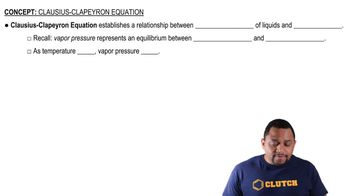00:59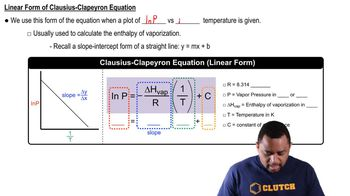02:57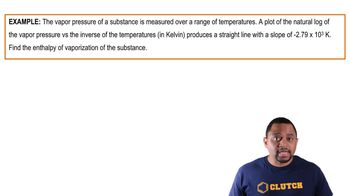01:37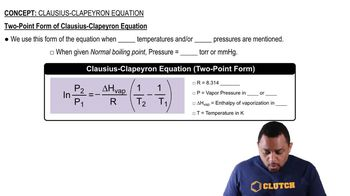01:13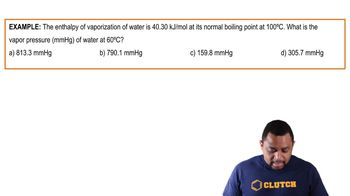03:37